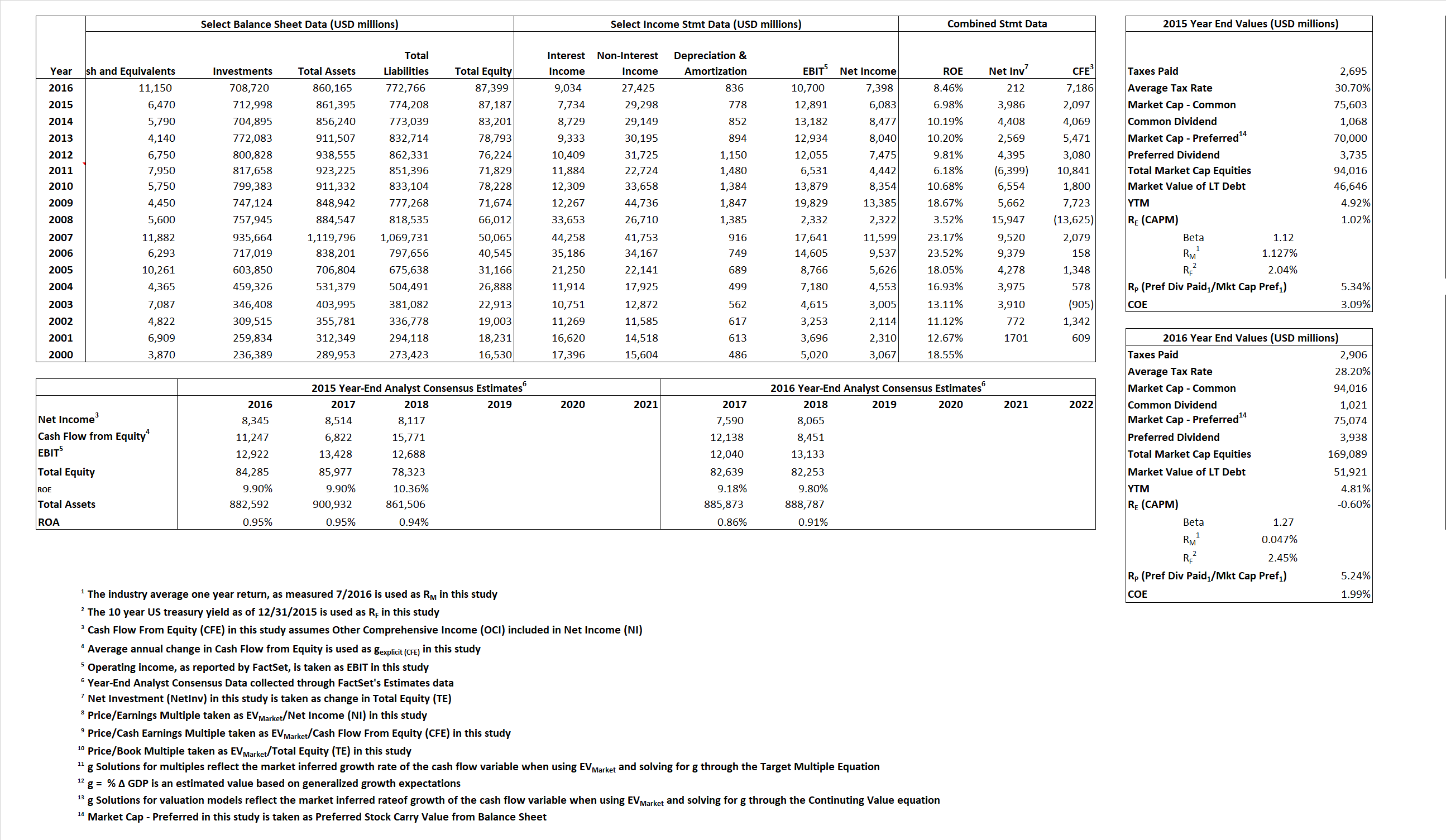# Goldman Sachs Group, Inc.

### Analyst Listings

The following analysts provide coverage for the subject firm as of May 2016:

 Broker Analyst Analyst Email Credit Suisse Christian Bolu christian.bolu@credit-suisse.com Buckingham Research James Mitchell jmitchell@buckresearch.com Nomura Research Steven Chubak Steven.Chubak@nomura.com Deutsche Bank Research Matthew O’Connor matthew.o-connor@db.com Oppenheimer Chris Kotowski chris.kotowski@opco.com Sandler O’Neill & Partners Jeffery J. Harte jharte@sandleroneill.com Evercore ISI Glenn Schorr glenn.schorr@evercoreisi.com Guggenheim Securities Eric Wasserstrom eric.wasserstrom@guggenheimpartners.com RBC Capital Markets Fiona Swaffield fiona.swaffield@rbccm.com Atlantic Equities Christopher Wheeler c.wheeler@atlantic-equities.com JMP Securities Devin Ryan dryan@jmpsecurities.com Wells Fargo Securities Matthew H. Burnell matt.burnell@wellsfargo.com Keefe Bruyette & Woods Brian Kleinhanzl bkleinhanzl@kbw.com BMO Capital Markets James Fotheringham james.fotheringham@bmo.com

### Primary Input Data### Derived Input Data

 Derived Input Label 2015 Value 2016 Value Equational Form Net Income NI 6,083 7,398$NI\, =\, EBIT\,\,-\,Interest\,\, Expense\,\, -\,\, Taxes\,\, Paid$ Cash Flow From Equity CFE 2,097 7,186$CFE\,=\,\,NI\,\,-\,\,\Delta\,\,TE\,\,+\,\,OCI$ Total Equity TE 87,187 87,399$TE\,=\,Total\,\,Assets\,\,-\,\,Total\,\,Long-Term\,\,Debt$ Return on Equity ROE 6.98% 8.46%$ROE\,=\,\frac { NI}{TE}$ Net Investment NetInv 3,986 212$NetInv\,=\,{ {TE}_{1}}-{{TE}_{0}}$ Investment Rate IR 65.53% 2.87%$IR\,=\,\frac {NetInv}{NI}$ Cost of Equity COE 1.02% -0.60%$COE \,=\,R_{F}\,\,+\,\,(R_{M}\,-\,R_{F})\beta$ Enterprise value EVMarket 87,546 157,939$EV\,=\,Market\,\,Cap\,\,Equity\,-\,Cash$ Long-Run Growth g = IR x ROIC 4.57% 0.24% Long-run growth rates of the income variable are used in the Continuing Value portion of the valuation models. g = %$\Delta$ GDP 2.50% 2.50%

 Equational Form Observed Value Single-stage multiple g solution Two-stage valuation model g solution 12/31/2015 12/31/2016 12/31/2015 12/31/2016 12/31/2015 12/31/2016 PRICE/EARNINGS$\frac {Price}{Book\,\,Value} \,= \,\frac{ROE\, -\, g}{ROE\,(COE\,-\,g)}$ PRICE/CASH EARNINGS$\frac {Price}{Cash\,\,Earnings} \,= \,\frac{ROE\, -\, g}{ROE\,(COE\,-\,g)}\,(\frac{NI}{CE})$ PRICE/BOOK VALUE$\frac {Price}{Book\,\, Value} \,= \,\frac{ROE\, -\, g}{ROIC\,(COE\,-\,g)}\,\,x\,\,ROE\,\,=\,\,\frac{ROE\,\,-\,\,g}{COE\,\,-\,\,g}$